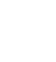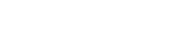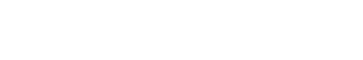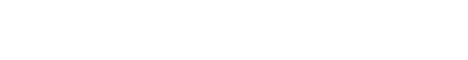Matrices on the ACT

Lots of students have minor panic attacks when they are first faced with matrices on the ACT Math Test, but never fear! Even if matrices look completely foreign to you, a quick read through this blog will calm your nerves. 🙂

Luckily, matrix questions are quite rare on the ACT (you might not see a single one throughout the 60 questions on the ACT Math Test!), and require only basic addition and subtraction skills (or *cough* a TI-83 calculator *cough*) to solve. If you can add 5 and 6, you’ll be fine!

ACT Matrix Basics

The word matrix refers to a rectangular-looking box filled with numbers arranged in rows and columns Each number in the matrix is called an element. When it comes to matrices on the test, you really just need to know the basics:

First of All, Matrices Are Referred to by Their Dimensions.

Matrices can come in all sorts of sizes. We write the size of a matrix first with its number of rows and then its number of columns.

This, for example, is a 3 x 2 matrix. 3 rows and 2 columns:Scalar Multiplication

You might see a question on the ACT that asks you do what is called scalar multiplication. This means that we are multiplying a matrix by an ordinary number. Every entry inside the matrix just gets multiplied by that number.You might also see a question on the ACT on adding or subtracting matrices. Important note: you can only add or subtract two matrices of exactly the same dimensions. We add or subtract matrices by adding or subtracting the corresponding numbers (the numbers that are in the same “spot” on each matrix).Notice that the size of the matrices is the same, and that each element in the first matrix is added to the corresponding element in the second matrix to get the corresponding element in the third matrix. -3 + -2 = -5.

With that understanding, we can solve for x by writing a simple equation: x + 9 = 11; x = 2.

Multiplying Matrices

All right, I saved the trickiest for last. You can only multiply matrices if the number of columns in first matrix equals the number of rows in the second matrix. When we multiply matrices, the product matrix will have the same number of rows as first matrix and the same number of columns as the second. For example, the product of a 2 x 3 matrix and a 3 x 2 matrix would be a 3 x 3 matrix.In order to find the entry in the first column, first row of the product matrix, we are going to multiply each number in the first row of the first matrix by its corresponding number in the first column of the second matrix and add the products together.For the entry in the second row, second column, we will do the same thing with the second row of the first matrix and the second column of the second matrix:In order to completely fill out this matrix, we would have to do 9 calculations, but don’t fret, the ACT is more likely to give you smaller matrices or matrices with more zeros or ask you to find just one entry in a matrix.

Let’s look at a question involving subtracting matrices.

Guided ACT Matrix Practice QuestionWhat is the sum of x and y?

To answer this, we will set up two equations to solve for x and y independently.

16 – 2 = x and 3 – (-4) = y

14 = x and 7 = y

Their sum is 14 + 7 = 21.

Sometimes a variable will be introduced to stand for an entire matrix. Let’s look at an example:Carefully subtract each corresponding element.Now try a practice question on your own!

More ACT Matrix Practice QuestionsWhat is the solution to the matrices?To subtract matrices, subtract each corresponding element, as shown above. Remember that subtracting a negative number becomes addition.

With practice, you’ll see that the math involved in ACT matrix questions is rarely challenging – just keep the rows and columns lined up and you can’t help but get them right!

Author

•Mike served as a GMAT Expert at Magoosh, helping create hundreds of lesson videos and practice questions to help guide GMAT students to success. He was also featured as "member of the month" for over two years at GMAT Club. Mike holds an A.B. in Physics (graduating magna cum laude) and an M.T.S. in Religions of the World, both from Harvard. Beyond standardized testing, Mike has over 20 years of both private and public high school teaching experience specializing in math and physics. In his free time, Mike likes smashing foosballs into orbit, and despite having no obvious cranial deficiency, he insists on rooting for the NY Mets. Learn more about the GMAT through Mike's Youtube video explanations and resources like What is a Good GMAT Score? and the GMAT Diagnostic Test.